## Time Series Analysis - Forecasting and Control

A time series is a sequence of observations taken sequentially in time.

#### Chapter 1 - Introduction

Exercise 1.1: The dataset airquality in the R datasets package includes information on daily air quality measurements in New York, May to September 1973. The variables included are mean ozone levels at Roosevelt Island, solar radiation at Central Park, average wind speed at LaGuardia Airport, and maximum daily temperature at LaGuardia Airport; see help(airquality) for details.

(a) Load the dataset into R.

(b) Investigate the structure of the dataset.

(c) Plot each of the four series mentioned above using the plot() command in R; see help(plot) for details and examples.

(d) Comment on the behavior of the four series. Do you see any issues that may require special attention in developing a time series model for each of the four series.

In :
``````import pandas as pd
import numpy as np
import matplotlib
import matplotlib.pyplot as plt
matplotlib.rcParams.update({'font.size': 10})``````
In [ ]:
``````import jovian
!jovian version
jovian.commit(files=['airquality.csv', 'AirPassengers.csv', 'ORCL.csv', 'data'], nb_filename='Time Series Analysis - Forecasting and Control.ipynb')``````
```Jovian, version 0.1.90.dev4 [jovian] Saving notebook.. ```
In :
``````path_to_dataset = 'airquality.csv'
data = pd.read_csv(path_to_dataset, index_col=0)``````
In :
``data.head()``
Out:
In :
``````variables = [x for x in data.columns if x not in ['Month', 'Day']]
for col in variables:
fig = plt.figure()
plt.plot(data[col])
plt.ylabel(col, fontsize=18)
plt.xlabel('time', fontsize=16)``````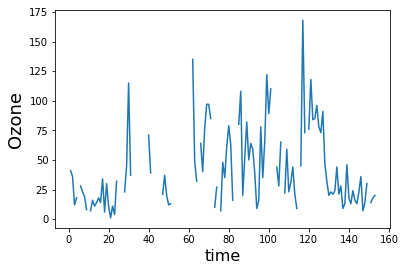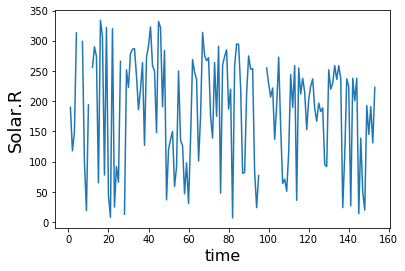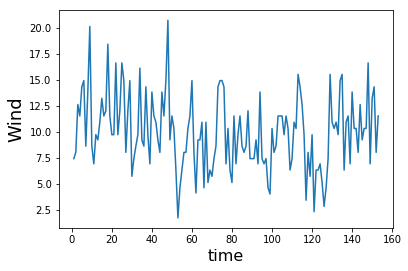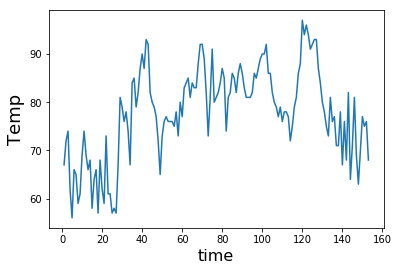In :
``````print('Standard Deviations')
df_std = data.describe().loc['std', :].sort_values()
df_std.loc[variables]
``````
```Standard Deviations ```
Out:
``````Ozone      32.987885
Solar.R    90.058422
Wind        3.523001
Temp        9.465270
Name: std, dtype: float64``````

Comment on the behavior of the four series. Do you see any issues that may require special attention in developing a time series model for each of the four series.

all of the plots are nonstationary

Exercise 1.2: Monthly totals of international airline passengers (in thousands of passengers), January 1949--December 1960, are available as Series G in Part Five of this book. The data are also available as series AirPassengers in the R datasets package.

(a) Load the dataset into R and examine the structure of the data.
(b) Plot the data using R and describe the behavior of the series.
(c) Perform a log transformation of the data and plot the resulting series. Compare the behavior of the original and log-transformed series. Do you see an advantage in using a log transformation for modeling purposes?

In :
``path_to_dataset_2 = 'AirPassengers.csv'``
In :
``data = pd.read_csv(path_to_dataset_2)``
In :
``````fig, (ax1, ax2) = plt.subplots(1, 2, figsize=(20,5))

ax1.set_ylabel('Passengers', fontsize=20)
ax1.plot(data['Passengers'])

ax2.set_ylabel('log(Passengers)', fontsize=20)
ax2.plot(np.log(data['Passengers']), 'r')``````
Out:
``[<matplotlib.lines.Line2D at 0x11cad64e0>]``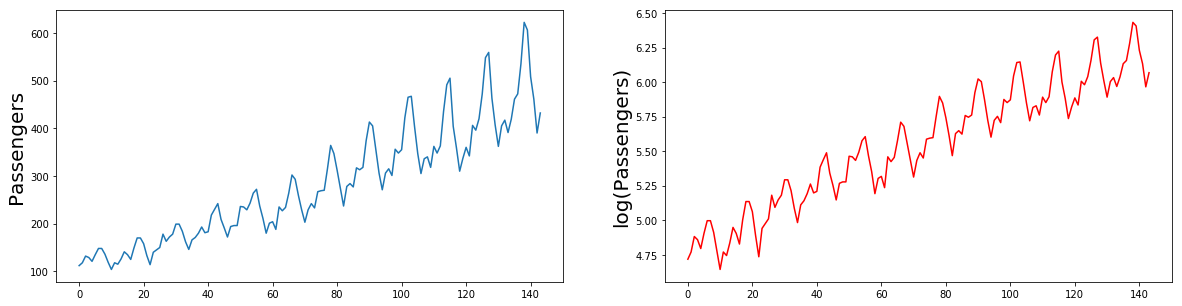Perform a log transformation of the data and plot the resulting series. Compare the behavior of the original and log-transformed series. Do you see an advantage in using a log transformation for modeling purposes?

Mean and variance across intervals remains constant (?) - Not sure

Exercise 1.3: Download a time series of your choosing from the Internet. Note that financial and economic time series are available from sources such as Google Finance and the Fed- eral Reserve Economic Data (FRED) of Federal Reserve Bank in St. Louis, Missouri, while climate data is available from from NOAA’s National Climatic Data Center (NCDC).
(a) Store the data in a text file or a .csv file and read the data into R.
(b) Examinethepropertiesofyourseriesusingplotsorotherappropriatetools.
(c) Does your time series appear to be stationary? If not, would differencing and/or some other transformation make the series stationary?

Let us look at Oracle Corporation (ORCL) data since it's IPO in 1986.

In :
``````data_orcl = pd.read_csv('ORCL.csv', index_col=0)
data_orcl['Adj Close'].plot(figsize=(20,6))
plt.title('ORCL Adj Close', fontsize=25)
_ = plt.xlabel('Date', fontsize=20)``````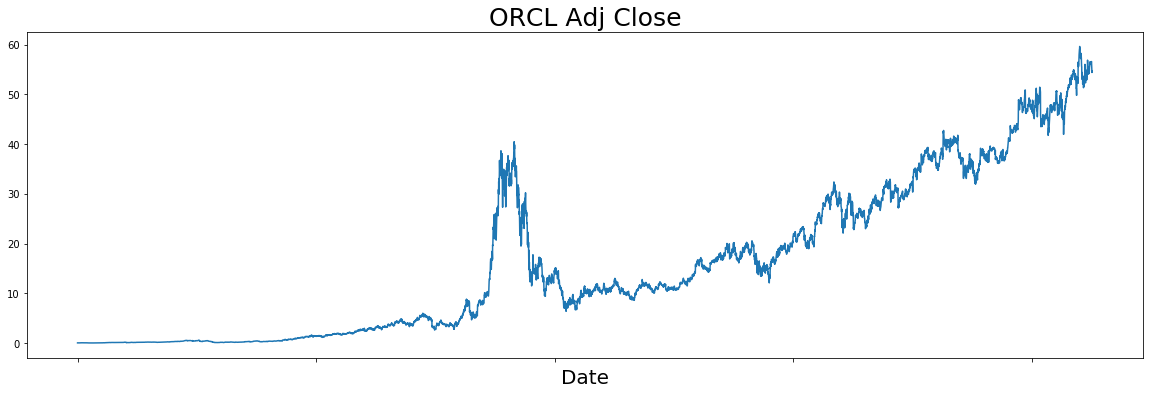## Part one - Stochastic models and their forecasting

A model that describes the probability structure of a sequence of observations is called a stochastic process.

An important class of stochastic processes discussed in Chapter 2 is the stationary processes. They are assumed to be in a specific form of statistical equilibrium, and in particular, vary over time in a stable manner about a fixed mean

Particular stationary stochastic processes of value in modeling time series are the autore- gressive (AR), moving average (MA), and mixed autoregressive--moving average (ARMA) processes.

Because many practically occurring time series (e.g., stock prices and sales figures) have nonstationary characteristics, the stationary models introduced in Chapter 3 are developed further in Chapter 4 to give a useful class of nonstationary processes called autoregressive integrated moving-average (ARIMA) models.

#### Chapter 2 - Autocorrelation function and spectrum of stationary processes

A central feature in the development of time series models is an assumption of some form of statistical equilibrium.

Usually, a stationary time series can be usefully described by its mean, variance, and autocorrelation function or equivalently by its mean, variance, and spectral density function

In :
``from sklearn.linear_model import LinearRegression``
In :
``````model = LinearRegression()

def best_fit_line(x, y):
model.fit(x, y)
return (x, model.predict(x))

def plot_auto_covariance(data, col_name):
fig, (ax1, ax2) = plt.subplots(1, 2, figsize=(20,5), constrained_layout=True)
fig.suptitle(col_name)
y1 = data[2:70]
x1 = data[1:69]

y2 = data[10:77]
x2 = data[1:68]

ax1.scatter(x1, y1)
x, y = best_fit_line(x1.values.reshape(-1, 1), y1.values.reshape(-1, 1))
ax1.plot(x, y, 'g')
ax1.set_title('Scatter plot of lag k = 1', fontsize=20)
ax1.set_xlabel('z[t]')
ax1.set_ylabel('z[t + 1]')

ax2.scatter(x2, y2, c='r')
x, y = best_fit_line(x2.values.reshape(-1, 1), y2.values.reshape(-1, 1))
ax2.plot(x, y, 'g')
ax2.set_xlabel('z[t]')
ax2.set_ylabel('z[t + 9]')
ax2.set_title('Scatter plot of lag k = 9', fontsize=20)``````

Let us look at the covariance plots of some datasets

In :
``````path_to_dataset_2 = 'AirPassengers.csv'
data = pd.read_csv(path_to_dataset_2)
data = data['Passengers']
plot_auto_covariance(data, 'Passengers')``````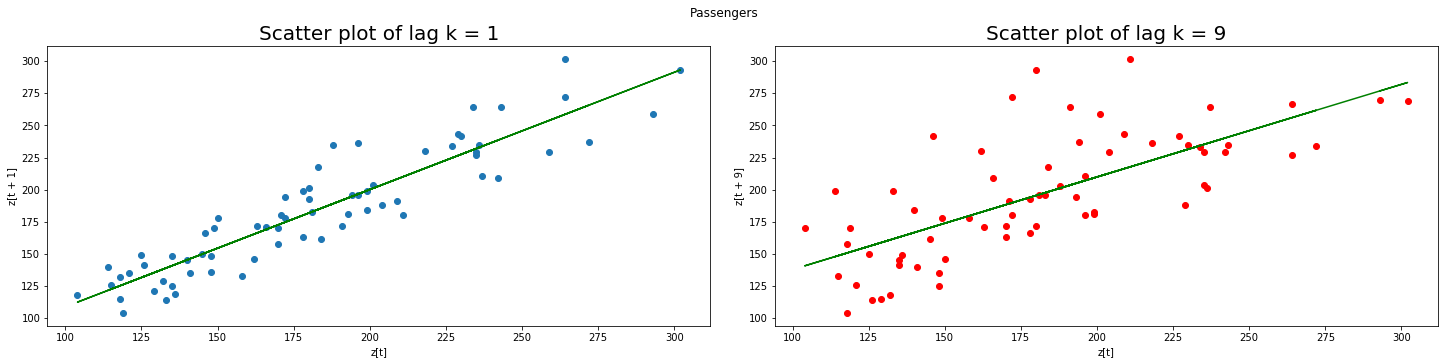In :
``````path_to_dataset = 'airquality.csv'
data = pd.read_csv(path_to_dataset, index_col=0)
variables = [x for x in data.columns if x not in ['Month', 'Day']]
data = data.dropna()
for var in variables:
plot_auto_covariance(data[var], var)
``````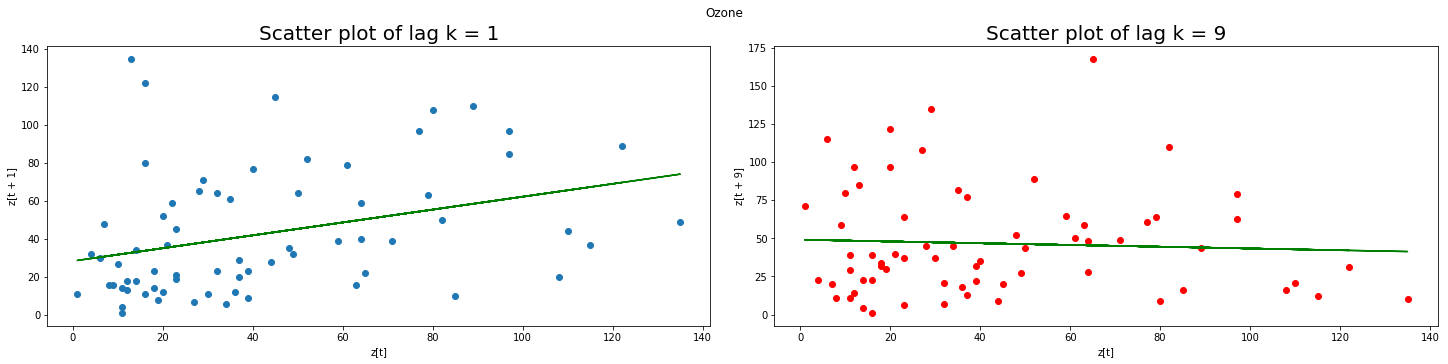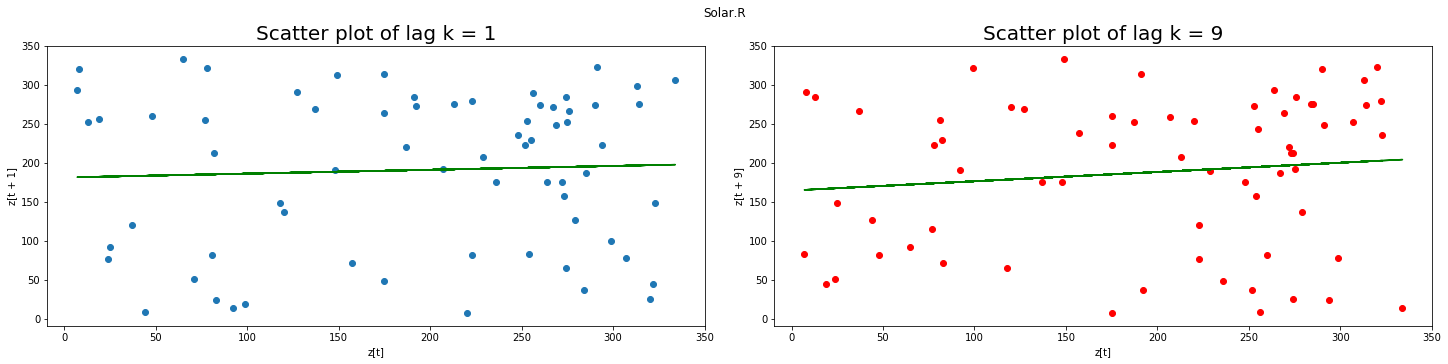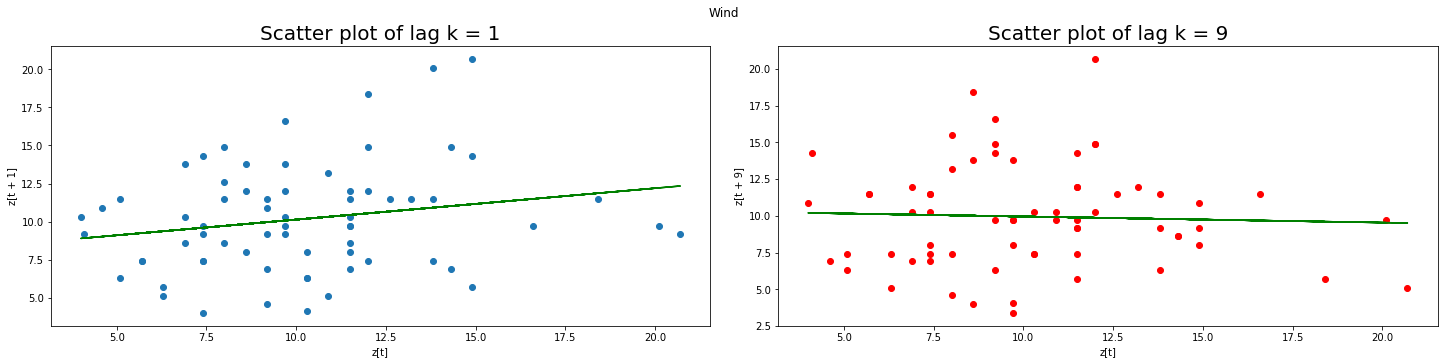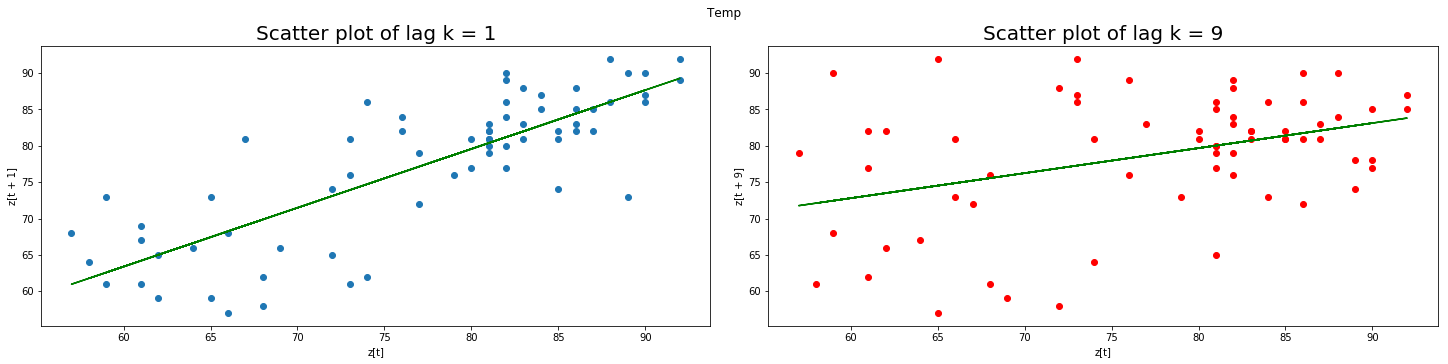In :
``from statsmodels.tsa.stattools import acf``
In :
``````path_to_dataset_2 = 'AirPassengers.csv'
data = pd.read_csv(path_to_dataset_2)
data = data['Passengers']

plt.stem(acf(data, 3, fft=True))
plt.title('Autocorrelation function')``````
Out:
``Text(0.5, 1.0, 'Autocorrelation function')``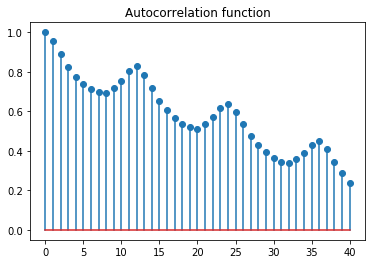In [ ]:
``````path_to_dataset = 'airquality.csv'
data = pd.read_csv(path_to_dataset, index_col=0)
variables = [x for x in data.columns if x not in ['Month', 'Day']]

fig, axs = plt.subplots(nrows=2, ncols=2, figsize=(15, 6), sharex=True, sharey=True)

data = data.dropna()
for var, ax in zip(variables, axs.flat):
ax.stem(acf(data[var], 3, fft=True))
ax.set_title('Autocorrelation function of {}'.format(var))
ax.set_ylim(-0.5, 1)
plt.tight_layout()
plt.show()
``````
In :
``print('Hello world')``
```Hello world ```
In :
``!pip install jovian``
```Requirement already satisfied: jovian in /Users/rohitsanjay/miniconda3/lib/python3.7/site-packages (0.1.89) Requirement already satisfied: uuid in /Users/rohitsanjay/miniconda3/lib/python3.7/site-packages (from jovian) (1.30) Requirement already satisfied: requests in /Users/rohitsanjay/miniconda3/lib/python3.7/site-packages (from jovian) (2.22.0) Requirement already satisfied: pyyaml in /Users/rohitsanjay/miniconda3/lib/python3.7/site-packages (from jovian) (5.1.2) Requirement already satisfied: certifi>=2017.4.17 in /Users/rohitsanjay/miniconda3/lib/python3.7/site-packages (from requests->jovian) (2019.9.11) Requirement already satisfied: chardet<3.1.0,>=3.0.2 in /Users/rohitsanjay/miniconda3/lib/python3.7/site-packages (from requests->jovian) (3.0.4) Requirement already satisfied: urllib3!=1.25.0,!=1.25.1,<1.26,>=1.21.1 in /Users/rohitsanjay/miniconda3/lib/python3.7/site-packages (from requests->jovian) (1.24.2) Requirement already satisfied: idna<2.9,>=2.5 in /Users/rohitsanjay/miniconda3/lib/python3.7/site-packages (from requests->jovian) (2.8) ```
In :
``jovian.commit(files=['airquality.csv', 'AirPassengers.csv'])``
```[jovian] Saving notebook.. ```
```[jovian] Updating notebook "748724f73cef4353ae5c7ed3098bcbcf" on https://jovian.ml/ [jovian] Uploading notebook.. [jovian] Capturing environment.. [jovian] Uploading additional files.. [jovian] Committed successfully! https://jovian.ml/rohit/timeseries ```
In :
``jovian.log_metrics``
Out:
``<function jovian.log_metrics(data, verbose=True)>``
In :
``help(jovian.log_metrics)``
```Help on function log_metrics in module jovian: log_metrics(data, verbose=True) Record metrics for the current experiment Args: data(dict): A python dict or a array of dicts to be recorded as metrics. verbose(bool, optional): By default it prints the acknowledgement, you can remove this by setting the argument to False. Example .. code-block:: import jovian metrics = { 'epoch': 1, 'train_loss': .5, 'val_loss': .3, 'acc': .94 } jovian.log_metrics(metrics) ```
In :
``````jovian.log_metrics({
'epoch' : 100,
'train_loss' : 0.06,
'val_loss' : 0.01,
'acc' : 0.95
})
``````
```[jovian] Metrics logged. ```
In :
``!touch model.h5``
In :
``!touch model.pkl``
In [ ]:
``jovian.commit(artifacts=['model.h5', 'model.pkl'])``
```[jovian] Saving notebook.. ```
In [ ]:
`` ``